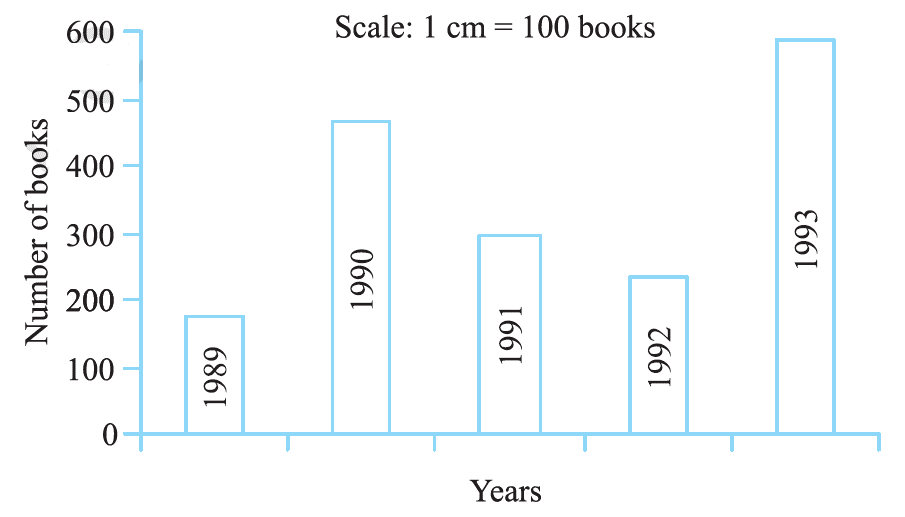# Ex.3.3 Q2 Data Handling - NCERT Maths Class 7

## Question

Use the bar graph (see below figure) which shows the no. of books sold by a bookstore during $$5$$ consecutive years and answer the following questions:

(i) About how many books were sold in $$1989, 1990, \text &\,\, 1992 ?$$

(ii) In which year was about $$475$$ books sold? About $$225$$ books sold?

(iii) In which years were fewer than $$250$$ books sold?

(iv) Can you explain how you would estimate the number of books sold in $$1989?$$Video Solution
Data Handling
Ex 3.3 | Question 2

## Text Solution

What is known?

Number of books sold during five consecutive years.

Reasoning:

We can answer all the question by observing the bar graph

Steps:

From the given bar graph, we have

(i) In $$1989 ,180$$ books were sold.

• In $$1990, 475$$ books were sold.
• In $$1992, 225$$ books were sold.

(ii) In $$1990$$ about $$475$$ books were sold and in $$1992$$ about $$225$$ books were sold.

• In $$1989$$ and $$1992$$ fewer than $$250$$ books were sold.
• From the graph we can conclude that $$180$$ books were sold in $$1989.$$
Learn from the best math teachers and top your exams

• Live one on one classroom and doubt clearing
• Practice worksheets in and after class for conceptual clarity
• Personalized curriculum to keep up with school